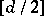# Nilpotent algebra

(diff) ← Older revision | Latest revision (diff) | Newer revision → (diff)
An algebra for which there is a natural numbersuch that any product ofelements of the algebra is zero. If there is a non-zero product ofelements, thenis called the index of nilpotency of the algebra. Examples of nilpotent algebras are: an algebra with zero multiplication; an algebra of strictly upper-triangular matrices; direct sums of nilpotent algebras the indices of nilpotency of which are uniformly bounded; and the tensor product of two algebras one of which is nilpotent.
The class of nilpotent algebras is closed under taking homomorphic images and subalgebras. In an associative algebra (cf. Associative rings and algebras) the sum of finitely many nilpotent ideals is a nilpotent ideal, and the sum of an arbitrary set of nilpotent ideals is, generally speaking, locally nilpotent. A finite-dimensional algebra over a field of characteristic zero having a basis consisting of nilpotent elements is nilpotent. If an algebra satisfies a polynomial identity of degree, then every nilpotent subring of it of degreebelongs to a sum of nilpotent ideals. The derived algebra of a finite-dimensional Lie algebra over a field of characteristic zero is nilpotent. Nilpotent subalgebras that coincide with their normalizer (Cartan subalgebras) play an essential role in the classification of simple Lie algebras of finite dimension. A nilpotent Lie algebra has an outer automorphism. A Lie algebra with a regular automorphism (that is, one having no fixed point except zero) of prime period is nilpotent.